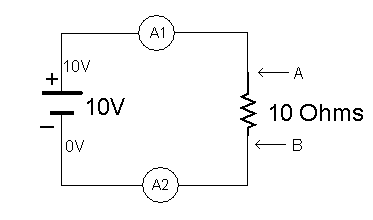# Relationship between voltage, power and current

Misplaced homework thread moved here by moderator, hence no template.
Hy,
If we double the voltage than what will be double
A. power
B. Current
C. Resistance
D. Both a & b

Svein
As usual: It depends. You have not specified the context. A drawing would help.

As usual: It depends. You have not specified the context. A drawing would help.
When we double the voltage in a simple electric circuit, we double the
A. Current
B. Power
C. Resistance
D. Both current and power

davenn
Gold Member
When we double the voltage in a simple electric circuit, we double the

you still haven't specified the circuit setup
until you fix (state) a couple of the variables, your Q cannot be answered

Dave

you still haven't specified the circuit setup
until you fix (state) a couple of the variables, your Q cannot be answered

Dave
Actually problem is that
This question is on the text book which is recommended by government.

Actually problem is that
This question is on the text book which is recommended by government.
So there is not show any circuit

davenn
Gold Member
So there is not show any circuit

so, so let me provide you with a circuit ......a nice simple one, a battery and a resistor

do you know Ohm's Law ? ....

so, so let me provide you with a circuit ......

View attachment 111995

a nice simple one a battery and a resistor

do you know Ohm's Law ? ....
Yes I know

davenn
Gold Member
Yes I know

OK, good

so you have 10V across a 10 Ohm resistor ...
what is the current flowing in that circuit ?

show me your workingOK, good

so you have 10V across a 10 Ohm resistor ...
what is the current flowing in that circuit ?

show me your working1 A

davenn
Gold Member
1 A

correct

so if you now increase the voltage to 20 V, what is the current in the circuit ?

correct

so if you now increase the voltage to 20 V, what is the current in the circuit ?
2 A

davenn
Gold Member
2 A

correctso next, what is the formula for finding power ?

correct [emoji2]
so next, what is the formula for finding power ?
P = I V
P= I (2) R
P = V (2) / R

davenn
Gold Member
P = I V
P= I (2) R
P = V (2) / R

Yup
for ease we can use your first choice P = I x V

work out the two power levels for the examples we have just done

Hy,
If we double the voltage than what will be double
A. power
B. Current
C. Resistance
D. Both a & b

Dave

Yup
for ease we can use your first choice P = I x V

work out the two power levels for the examples we have just done

Dave
Mean D option is correct

davenn
Gold Member
Mean D option is correct

yes, well donewasn't too difficult to work out, huh.

Dave

yes, well done [emoji2]
wasn't too difficult to work out, huh.

Dave
Thanks
But
Is this answer corrected. If current is double
P = 2 I * 2 V

davenn
Gold Member
Is this answer corrected. If current is double

unlike voltage or resistance, current cannot just change
current is a RESULT of a given voltage across a given resistance
That is, current can ONLY change IF the voltage OR the resistance changes

And the power, in Watts, dissipated in a circuit, in our circuits above ( in the resistor)
is always related to the voltage across the resistance divided by the resistance

so for a fixed resistance, if the voltage is doubled, then the current is doubled and therefore so is the power dissipated

Dave

unlike voltage or resistance, current cannot just change
current is a RESULT of a given voltage across a given resistance
That is, current can ONLY change IF the voltage OR the resistance changes

And the power, in Watts, dissipated in a circuit, in our circuits above ( in the resistor)
is always related to the voltage across the resistance divided by the resistance

so for a fixed resistance, if the voltage is doubled, then the current is doubled and therefore so is the power dissipated

Dave
Power dissipated mean?

Mean energy loss

•davenn
davenn
Gold Member
Power dissipated mean?

power lost, radiated, used up <---- take your pick, all the samepower lost, radiated, used up <---- take your pick, all the same[emoji3]

fresh_42
Mentor
I moved it to homework, where it better fits and can be found by others with similar questions.

gneill
Mentor
Mean D option is correct
Check again the amount by which current and power changed. They don't change by the same factor.

•conscience, Merlin3189 and davenn